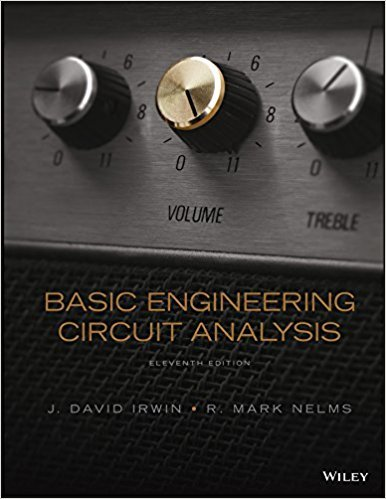×
Get Full Access to Basic Engineering Circuit Analysis - 11 Edition - Chapter 5 - Problem 5.6
Get Full Access to Basic Engineering Circuit Analysis - 11 Edition - Chapter 5 - Problem 5.6

×

# Find Io in the network in Fig. P5.6 using superposition. 6ISBN: 9781118539293 159

## Solution for problem 5.6 Chapter 5

Basic Engineering Circuit Analysis | 11th Edition

• Textbook Solutions
• 2901 Step-by-step solutions solved by professors and subject experts
• Get 24/7 help from StudySoup virtual teaching assistantsBasic Engineering Circuit Analysis | 11th Edition

4 5 1 241 Reviews
30
1
Problem 5.6

Find Io in the network in Fig. P5.6 using superposition. 6 k 3 k 3 k Io 6 V + 2 k 2 mA Figure P5.6

Step-by-Step Solution:
Step 1 of 3

, p\:7 " ~ \If ~f11 ~ ...,., ~ » \ -: > ~'-1 ~ ~ I' tf YJ 1 -+- ~ ~

Step 2 of 3

Step 3 of 3

##### ISBN: 9781118539293

The answer to “Find Io in the network in Fig. P5.6 using superposition. 6 k 3 k 3 k Io 6 V + 2 k 2 mA Figure P5.6” is broken down into a number of easy to follow steps, and 26 words. This full solution covers the following key subjects: fig, figure, Find, Network, Superposition. This expansive textbook survival guide covers 15 chapters, and 1430 solutions. Since the solution to 5.6 from 5 chapter was answered, more than 507 students have viewed the full step-by-step answer. The full step-by-step solution to problem: 5.6 from chapter: 5 was answered by , our top Engineering and Tech solution expert on 11/23/17, 05:00AM. This textbook survival guide was created for the textbook: Basic Engineering Circuit Analysis, edition: 11. Basic Engineering Circuit Analysis was written by and is associated to the ISBN: 9781118539293.

Unlock Textbook Solution